# Cinema

Cinema auditorium is built for 3300 people. The first row is planned for 36 seats and each next gradually 4 more. How many rows of seats will have auditorium?

n =  33

### Step-by-step explanation:

Our quadratic equation calculator calculates it.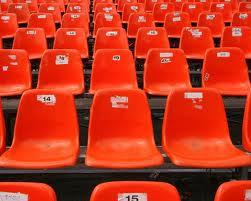Did you find an error or inaccuracy? Feel free to write us. Thank you!

Showing 1 comment:Sion
I like this questionTips to related online calculators
Looking for help with calculating roots of a quadratic equation?
Do you have a linear equation or system of equations and looking for its solution? Or do you have a quadratic equation?

## Related math problems and questions:

• Cinema 4In the cinema are 1656 seats and in the last row are 105 seats, in each next row 3 seats less. How many are the total rows in cinema?
• Cinema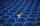How many rows of seats are in the stands, if you know that the first row has 24 seats and the last 50 seats and each subsequent row has two seats more than the row before? How many seats are in the stands?
• Chairs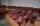Determine the number of seats in the seventh row and ninth row, if the 3rd row has 14 seats and in every next row of seats has five more than the previous row.
• SeatsSeats in the sport hall are organized so that each subsequent row has five more seats. First has 10 seats. How many seats are: a) in the eighth row b) in the eighteenth row
• Students at cinema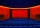All pupils from the school went to the cinema for a film performance. The cinema has 400 seats, 2 rows of 20 seats remain unoccupied. Teachers occupied 10 places. How many students were there?
• TheaterThe theater has in each row with 19 seats. Ticket to the first 10 rows is for 30 USD. In the next rows is for 15 USD. The theater was completely sold out. Revenue was 12255 USD. How many rows are in the theater?
• Cans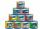How many cans must be put in the bottom row if we want 182 cans arrange in 13 rows above so that each subsequent row has always been one tin less? How many cans will be in the top row?
• Roof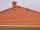Tiles are stacked in rows on the trapezoidal shaped roof. At the ridge is 15 tiles and each subsequent row has one more tile than in the previous row. How many tiled is covered roof if lowermost row has 37 tiles?
• Students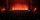In the front row sitting three students and in every other row 11 students more than the previous row. Determine how many students are in the room when the room is 9 lines, and determine how many students are in the seventh row.
• Built-up areaJohn build up area 5 x 7 = 35 m2 with building with a wall thickness 30 cm. How many centimeters would have to subtract from thickness of the walls that built-up area fell by 9%?
• To the cinemaJane at 8 am got message that all 1093 school pupils will go to the cinema. Within 20 min she said it to the three friends. Each of them again for 20 minutes said to the other three. In this way the message spread further. At what time all the children in
• Friends in cinema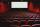5 friends went to the cinema. How many possible ways can sit in a row, if one of them wants to sit in the middle and the remaining's place does not matter?
• Geometric progressiobIf the sum of four consective terms of geometric progression is 80 and arithmetic mean of second and fourth term is 30 then find terms?
• Row for lunchThere are less than 66 people in a row for lunch. In front of Eva, there are 28 students. Behind Martin, there are 30 people. Between Eva and Martin, there are 17 people. How many students stand behind Eva if Martin stands in front of Eva?
• Tower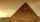Charles built a tower of cubes with an edge 2 cm long. In the lowest layer there were 6 cubes (in one row) in six rows, in each subsequent layer always 1 cube and one row less. What volume in cm3 did the whole tower have?
• In the cinema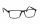There were 560 people in the cinema. 35% of them wore glasses. Of this number, 25% were women. How many men wore glasses?
• Salat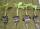Grandmother planted salad. In each row, he planted 13 seedlings. After a morning frost, many seedlings died. In the first row, five seedlings died. In the second row, two seedlings died more than 1st row. In the third row, three seedlings died less than 1